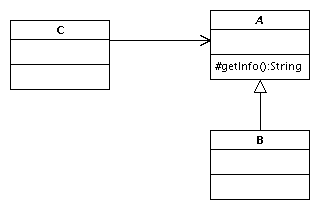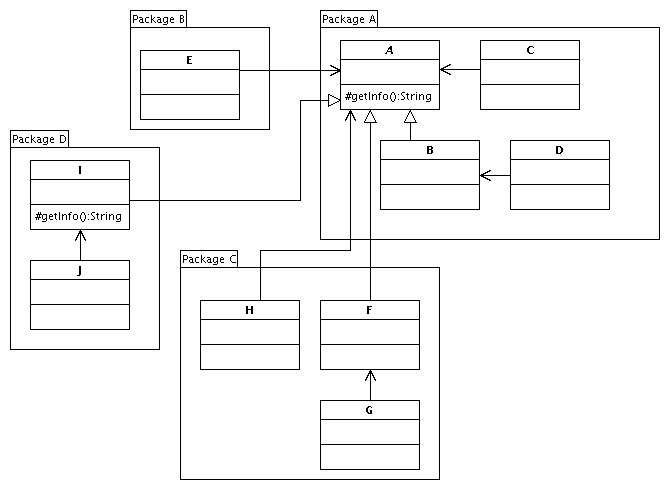eyt*
Aug 18, 2005

# protected in Java...

Here is an interesting question for Java developers. Consider the following classes, where class A is an abstract class that defines a protected method getInfo(), class B is a class that derives from A, and class C uses class A. In Java, is class C allowed to access the method getInfo()?The answer may surprise some, but the actual answer is that it depends, because I have omitted one important piece of information that is required to answer this question, and that is piece of information, as surprising as it may be, is what package do these classes belong to.

If you refer to section 6.6.1 of the Java Language Specification, you will note that the definition indicates that:

Otherwise, if the member or constructor is declared protected, then access is permitted only when one of the following is true:

• Access to the member or constructor occurs from within the package containing the class in which the protected member or constructor is declared.
• Access is correct as described in 6.6.2.

Of important note here is that classes within the same package as class A from the above example can access the method getInfo().

To drive this point home a bit more, consider the following cases:Here, we illustrate a Package A, which contains our abstract class A with the protected method getInfo(), class B which derives from class A, and class C and D which use class A and B, respectively. With this in mind, if class C instantiates an A and acquires information from it, it is OK.

• // From Package A, class C
• A a = new A();
• a.getInfo();
// OK

If class D does the same call via an object B, this is not valid:

• // From Package A, class D
• B b = new B();
• b.getInfo();
// OK

Now, if we do the above two calls in a separate package, this will not work. For example, in Package B, we have a class E, and should you attempt either of the above, this will result in a compilation error because now the two classes are not in the same package, and therefore, it is not accessible.

• // From Package B, class E
• A obj = new E();
• obj.getInfo();
// ERROR: We're not in the same package now!

In Package C, I show a new class F that is derived class from class A (from Package A); it does not, however, overload `getInfo()`. If class H instantiates an F via an A as follows, this will also result in an error since the compiler does not know that you are actually manipulating an F and thus treats this as an error.

• // From Package C, class H
• A obj = new F();
• obj.getInfo();
// ERROR: Maybe he compiler does not know A is really an F? Keep reading

But is that really the reason the compilation fails? Let's see. If we have another class G that accesses the F directly, you will still have a compilation error.

• // From Package C, class G
• F obj = new F();
• obj.getInfo();
// ERROR: F is not involved in getInfo()'s implementation

Interestingly enough, this is covered in the example in section 6.6.7, in which it states that this cannot be permitted since class G is not involved in the implementation of `A.getInfo()`.

So that brings us to Package D, which defines a class I that derives from class A and overrides the `getInfo()` method. Now in this package, if we try what we tried in the previous example in class J, we will note that it will now work. This is because class J is involved in the implementation of `getInfo`.

• // From Package D, class J
• J obj = new H();
• obj.getInfo();
// Works!

This is extremely interesting, especially if you come from a C++ background where packages do not exist. It is definitely an eye opener and will change my approach to class design!